# Proceedings of the Institute of Acoustics Novel Digital Techniques for Echo Cancellation Applied to Speech Signals

 Page 2/4 Date 31.03.2018 Size 219.46 Kb. #45501
1   2   3   4

#### 2.2.1. Derivation of LMS Algorithm

The derivation of the LMS algorithm depends on both of the theories that were discussed earlier, the wiener solution and the steepest descent algorithm. Equation (4) is used to update the filter coefficients.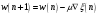(4)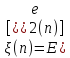Where:

Since the LMS algorithm operates based on the “random process implementation of the steepest descent algorithm – seen in Equation (5)”17, the error signal cannot be extracted. Therefore the immediate obtained value is used as an estimate.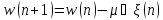(5)

Where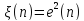By re-arrange Equation (4), and substituting it in Equation (4):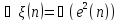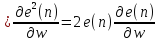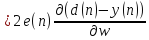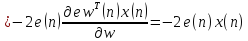(6)

Substituting (6) into (4) results in (7) which is the recursion of the LMS adaptive algorithm.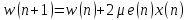(7)